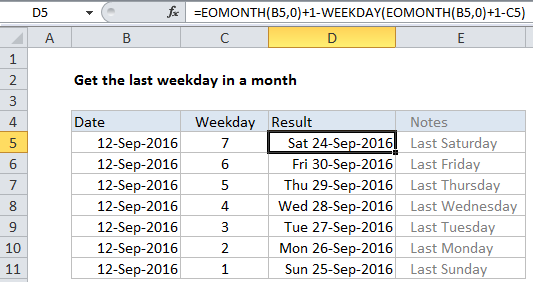## Excel Office

Excel How Tos, Tutorials, Tips & Tricks, Shortcuts

# Get last weekday in month in Excel

This tutorial shows you how to find the last weekday of the month in Excel.

To get the last weekday in a month (i.e. the last Saturday, the last Friday, the last Monday, etc) you can use a formula based on the EOMONTH and WEEKDAY functions.

## Formula

`=EOMONTH(date,0)+1-WEEKDAY(EOMONTH(date,0)+1-dow)`## Explanation

First, this formula determines the first day of the next month *after* a given date. It does this my using EOMONTH to get the last day of the month, then adding one day:

`=EOMONTH(B5,0)+1`

In the example shown, the formula in D5 is:

`=EOMONTH(B5,0)+1-WEEKDAY(EOMONTH(B5,0)+1-C5)`

Next, the formula calculates the number of days required to “roll back” to the last requested weekday in the month prior (i.e. the month of the original date):

`WEEKDAY(EOMONTH(B5,0)+1-C5)`

Inside WEEKDAY, EOMONTH is again used to get the first day of the next month. From this date, the value for day of week is subtracted, and the result is fed into WEEKDAY, which returns the number of days to roll back.

Worked Example:   Average response time per month in Excel

Last, the roll back days are subtracted from the first day of the next month, which yields the final result.

Worked Example:   EOMONTH function: Description, Usage, Syntax, Examples and Explanation

### Other weekdays

In the general form of the formula at the top of the page, day of week is abbreviated “dow”. This is a number between 1 (Sunday) and 7 (Saturday) which can be changed to get a different day of week. For example, to get the last Thursday of a month, set dow to 5.

Worked Example:   Calculate series of dates by workdays in Excel